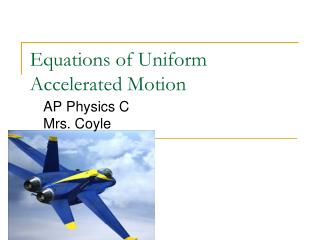DownloadDownload PresentationEquations of Uniform Accelerated Motion

# Equations of Uniform Accelerated Motion

Download Presentation## Equations of Uniform Accelerated Motion

- - - - - - - - - - - - - - - - - - - - - - - - - - - E N D - - - - - - - - - - - - - - - - - - - - - - - - - - -
##### Presentation Transcript

1. Equations of Uniform Accelerated Motion AP Physics CMrs. Coyle

2. Uniform Accelerated Motion • Motion with constant acceleration • Straight line • Same direction

3. Equations for Uniform Accelerated Motion • Velocity v= vo+ at • Position x= xo + vot + ½ at2 • v2 = vo2 + 2a(x-xo) Remember: Displacement= Dx = x-xo

4. MoreEquations of Motion for Uniform Accelerated Motion • vavg= ½ (vo+v) • Dx = ½ (vo+ v)t • Assume that ti=0

5. Example of Position vs Time (Positive Acceleration) Position (m) x= xo + vot + ½ at2 Parabola o Time (s) Slope of Tangent at a given time= Instantaneous Velocity at that time

6. Example of Velocity vs Time (Positive Acceleration) v= vo+ at Velocity (m/s) o Time (s) Slope of Line= Acceleration Area Under Line=Displacement

7. Example of Acceleration vs Time (Const. a) Acceleration (m/s2) o Time (s) Area under line = Change in Velocity

8. How do we derive Dx = ½ (vo+ v)t from the graph? v Velocity (m/s) vo o Time (s) t Hint: Area Under the Line=Displacement Δx

9. Example of Position vs Time (Negative Acceleration) Position (m) Parabola o Time (s)

10. Example of Velocity vs Time (Negative Acceleration) Velocity (m/s) o Time (s) Slope of Line= Acceleration Area Under Line=Displacement

11. Example of Acceleration vs Time (Negative a) Acceleration (m/s2) o Time (s) Area under line = Change in Velocity

12. How do we derive x= xo + vot + ½ at2? Hint: Start with Dx = ½ (vo+ v)t and then substitute for vthat v= vo+at.

13. How do we derive v2 = vo2 + 2a(x-xo)? • Hint: Start with Dx = ½ (vo+ v)t then substitute for t= (v– vo) /a

14. Problem 1 A ball initially stationary, accelerates at 0.25m/s2 down a 2m inclined plane. It then rolls up another incline, where it comes to rest after rolling up 1m. a) What is the speed of the ball at the bottom of the incline and how much time did this take? b) What is the acceleration along the second plane? Answer: a) 1m/s, 4sec, b) -0.5m/s2

15. Problem 2 A Mustang travelling with a constant velocity of 35m/s, passes a stationary police car. The reaction time of the officer was 2.5sec and he then accelerates at 5.0 m/s2 to catch the Mustang. How long does it take for the police car to catch the Mustang? Answer: 15.8sec